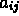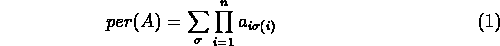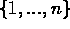Next: Interior Point Methods Up: Mathematical Algorithms and Previous: Object Oriented Numerical

Approximating the Permanent

Isabel Beichl, ACMD
Francis Sullivan, Supercomputing Research Center

The permanent of an nxn matrix with entriesis defined bywhere the sum is over all permutationsof. Informally, it similar to the determinant but without the alternating signs. However, in contrast with the determinant, no efficient procedure for computing this function is known. The permanent is important because it is equivalent to counting perfect matchings of a bipartite graph and arises naturally in a number of fields including discrete optimization, statistical physics and scheduling. Computing the permanent is typical of the kind of combinatorial problems that arise in data communications, a class of issues that NIST will need to face in coming years. Based on our experience in Monte Carlo simulation and in linear algebra we have developed an approximation algorithm that extends the best approximation algorithm for computing the permanent (Jerrum & Sinclair, SIAM J. Comput. v. 18) to a wider class of matrices. We use importance sampling as a method for reducing the variance of the samples generated and thus hasten convergence. We are in the process of developing a particularly efficient implementation based on some little known methods of linear algebra.